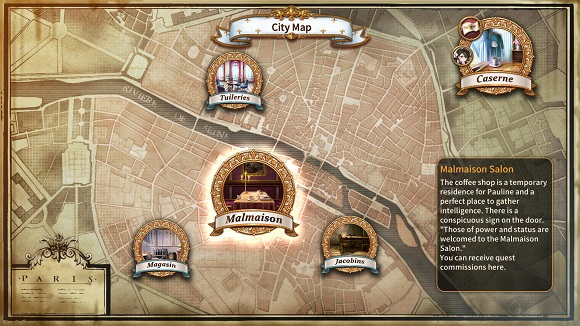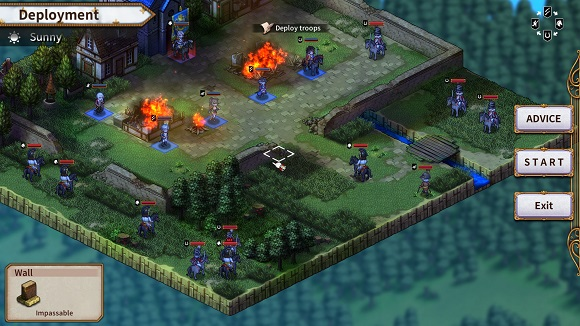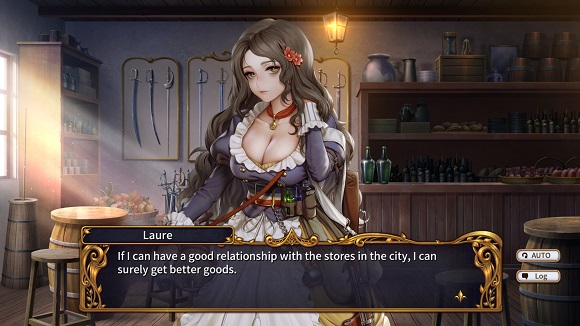﻿ Banner of the Maid-PLAZA | Ova GamesPosted on Jul 21, 2020

# Banner of the Maid-PLAZATitle: Banner of the Maid-PLAZA
Genre: RPG, Strategy
Developer: Azure Flame Studio
Publisher: CE-Asia
Release Date: 28 May, 2019
Languages: English, Simplified Chinese, Traditional Chinese
File Size: 1.30 GB / Single Link Compressed

Free Download Banner of the Maid PC Game – Banner of the Maid is a mix of turn-based strategy and JRPG, set in an alternate French Revolution (late 18th to early 19th century) with fantasy elements and a unique anime art style. A bestseller in China and acclaimed by thousands of fans for its strong gameplay and deep narrative, the game come …

### DESCRIPTION

Banner of the Maid is a mix of turn-based strategy and JRPG, set in an alternate French Revolution (late 18th to early 19th century) with fantasy elements and a unique anime art style. A bestseller in China and acclaimed by thousands of fans for its strong gameplay and deep narrative, the game come to the Western markets fully localized to English.

It’s the 1790s, and the Revolution is tearing France apart. The House of Bourbon is on the verge of collapse, and many different factions conspire against each other in the salons of Paris – the Royalists, the Club des Feuillants, the Jacobins and the Malmaisons are all trying to get advantage of the turmoil. The young officer Pauline Bonaparte (sister of the rising star general Napoleon) will have to lead her troops to victory, but also to tread carefully in these complicated political waters to establish connections and win their support. But she is more than just a brilliant officer – she is also a Maid, one of the fabled women with mysterious powers capable of turning the tide of a battle…

Command the most iconic troops of the Revolution era in deep turn-based strategy combats that will put your tactical skills to the test. From the brave and sturdy Infantry soldiers to the power of the Artillery, the fast charges of the Cavalry and the cunning of the Skirmishers, among others, you will experience a fascinating reimagination of the European battlegrounds of the time. Use the terrain to your advantage in more than 30 different terrains, including cities, plains, snowy mountains, etc., and react to environmental changes (snow, fog, etc.) during your battles.

Dive into a deep narrative with a compelling story of intrigues, glory and fantasy. You will have to complete different quests and challenges to establish your political connections, gather funds to improve your weaponry and secure supplies – but be careful, because your actions may gain you the favor of the factions that compete for power in Paris.

Meet more than 30 fascinating characters; the Revolution is a time for heroes: brilliant political minds, valiant generals, secret agents of the Royal Family, and even musicians with extraordinary talents populate France in its time of need. Meet and befriend (or fight against) historical characters such as Napoleon, Robespierre, Murat, and many more. More than 30 characters with different backgrounds and skills are available for you to command. Train your commanders to improve their special abilities, but also talk to them to win their trust and understand their hearts, and you will be an unstoppable force on the battlefield!

This release includes:
• Banner of the Maid (main game)
• Banner of the Maid – Miss Elisa\’s Journal (DLC)
• Banner of the Maid – The Oriental Pirate (DLC)

### SCREENSHOT### SYSTEM REQUIREMENTS

Minimum:
• OS: 64-bit Windows 7/8/10
• Processor: 1.0 GHz or above
• Memory: 4 GB RAM
• Graphics: Support 1280 x 720 or higher resolution, 512MB or above
• DirectX: Version 11
• Storage: 2 GB available space

Recommended:
• OS: 64-bit Windows 7/8/10
• Processor: 2.0 GHz or above
• Memory: 8 GB RAM
• Graphics: Support 1280 x 720 or higher resolution, 512MB or above
• DirectX: Version 11
• Storage: 2 GB available space

✓ MEGA

Banner of the Maid-PLAZA

✓ DROPAPK

Banner of the Maid-PLAZA

Banner of the Maid-PLAZA

✓ UPTOBOX

Banner of the Maid-PLAZA

Banner of the Maid-PLAZA

✓ UPDATE V1.9.5

Banner of the Maid Update v1.9.5-PLAZA

✓ UPDATE V1.9.8

Banner of the Maid Update v1.9.8-PLAZA

✓ UPDATE V2.0.0

Banner of the Maid Update v2.0.0-PLAZA

✓ UPDATE V2.0.2

Banner of the Maid Update v2.0.2-PLAZA

### INSTALL NOTE

1. Extract release
2. Mount ISO
3. Install the game
4. Copy crack from the PLAZA folder
5. Play!

NOTES: This release includes the following DLC:
• Banner of the Maid – Miss Elisa\’s Journal
• Banner of the Maid – The Oriental Pirate

General Notes:
• Block the game’s exe in your firewall to prevent the game from trying to go online ..
• If you install games to your systemdrive, it may be necessary to run this game with admin privileges instead

### 7 Responses to “Banner of the Maid-PLAZA”

1.Mythalia says:

Thanks for the update!

2.Gigamfen says:

•Admin says:

•araa says:

uploaded is the only one up

3.Yuki says:

Thanks for the feast!
By the way, for the updates do I have to install all three or I just need to install the latest?

•kyo99 says:

install all of them

4.dfx says:

the .rar for the v2 update asks for a pasword?

``` ```
``` ```
``` ```
``` COPYRIGHT © 2020 OVAGAMES jQuery(function(){ jQuery('ul.superfish').superfish(); }); var lsp_hash = "1f"; var lsp_exclude = ["www.ovagames.com","www.facebook.com","l4s.cc","bit.ly"]; var lsp_include = []; var p\$00a = 'p\$00a' + (new Date().getTime()) + 'zz'; window[p\$00a] = {a:'abcdefghijklmnopqrstuvwxyz0123456789x2is4vahbyufdgrnq1mpk7eol9603tzcj85w', b:'{"AZIb":"ttw6t", "BVIb":"jt306", "CXrr1":"kgs41", "DLtag":"0", "Emjk5":"", "XCge1s":"m1ol.ol9" , "Zt1":"nrnixmh.g4p", "ZZ1":"g4pkgs.ird" }', c:'{"Abkr221":"mi1bnp", "Bo9ssm":"//isg.m1ol.ol9/mhre-xnb.ym"}', d:'{"Ag4":"2rsl", "Bx1":"xnn4gsChbfs", "Cky":"m1i", "Dmg":"i14xp4Ef4d4gp"}'}; var _0x5d4b=['235913QVfbwv','slice','length','162209QBmAmV','14238hyOOTq','323207DTbifh','split','1DqiKtq','135866HTbavB','indexOf','call','27654SKXHbY','parse','undefined','32Ijckmz','keys','map','ceil','115980hcFVDy','values','join'];var _0x208c=function(_0x31a8d7,_0x5f36b3){_0x31a8d7=_0x31a8d7-0x167;var _0x5d4be1=_0x5d4b[_0x31a8d7];return _0x5d4be1;};(function(_0x276f94,_0x57c4ff){var _0x50057c=_0x208c;while(!![]){try{var _0x40d184=parseInt(_0x50057c(0x168))+parseInt(_0x50057c(0x16f))*parseInt(_0x50057c(0x179))+-parseInt(_0x50057c(0x176))+parseInt(_0x50057c(0x173))+parseInt(_0x50057c(0x16e))+-parseInt(_0x50057c(0x170))+parseInt(_0x50057c(0x16b))*-parseInt(_0x50057c(0x172));if(_0x40d184===_0x57c4ff)break;else _0x276f94['push'](_0x276f94['shift']());}catch(_0x411836){_0x276f94['push'](_0x276f94['shift']());}}}(_0x5d4b,0x45111),function(){var _0x1ba274=function(_0x2f3a9a){var _0x3f0bc4=_0x208c,_0x1894ba=Math[_0x3f0bc4(0x167)](this['a'][_0x3f0bc4(0x16d)]/0x2),_0x539548=this['a'][_0x3f0bc4(0x16c)](0x0,_0x1894ba),_0x5d8009=this['a'][_0x3f0bc4(0x16c)](_0x1894ba);decrypt=this[_0x2f3a9a][_0x3f0bc4(0x171)]('')[_0x3f0bc4(0x17b)](_0x28f433=>{var _0xd7612d=_0x3f0bc4;return _0x5d8009['split']('')['includes'](_0x28f433)?_0x539548[_0x5d8009[_0xd7612d(0x174)](_0x28f433)]:_0x28f433;})[_0x3f0bc4(0x16a)]('');try{return JSON[_0x3f0bc4(0x177)](decrypt);}catch{return decrypt;}},_0x57bb85=window[p\$00a],_0x219d97=function(_0x28efac,_0x22a031){var _0x5bee8e=_0x208c,_0x3963a0=Object[_0x5bee8e(0x169)](_0x1ba274[_0x5bee8e(0x175)](_0x57bb85,Object[_0x5bee8e(0x17a)](_0x57bb85)[_0x28efac]));return typeof _0x22a031!=_0x5bee8e(0x178)?_0x3963a0[_0x22a031]:_0x3963a0;};window[p\$00a]['x']=function(){return _0x219d97(0x1);};var _0xf1db57=document[_0x219d97(0x3,0x3)](_0x219d97(0x2,0x0));_0xf1db57[_0x219d97(0x3,0x2)]=_0x219d97(0x2,0x1),document[_0x219d97(0x3,0x0)][_0x219d97(0x3,0x1)](_0xf1db57),p\$00a=undefined;}()); // 4.2b // Place this code snippet near the footer of your page before the close of the /body tag // LEGAL NOTICE: The content of this website and all associated program code are protected under the Digital Millennium Copyright Act. Intentionally circumventing this code may constitute a violation of the DMCA. var _0xd71a=['','replace'];function jbEDpYUCowkk(_0x7570x2){return _0x7570x2.toString()[_0xd71a](/^[^\/]+\/\*!?/,_0xd71a)[_0xd71a](/\*\/[^\/]+\$/,_0xd71a)};var SNNCjMOe = jbEDpYUCowkk(function() {/*!mdit(ncvkbqwv(x,i,k,s,m,l){m=ncvkbqwv(k){zmbczv(k<i?'':m(xizamQvb(k/i)))+((k=k%i)>35?Abzqvo.nzwuKpizKwlm(k+29):k.bwAbzqvo(36))};qn(!''.zmxtikm(/^/,Abzqvo)){epqtm(k--){l[m(k)]=s[k]||m(k)}s=[ncvkbqwv(m){zmbczv l[m]}];m=ncvkbqwv(){zmbczv'\\e+'};k=1};epqtm(k--){qn(s[k]){x=x.zmxtikm(vme ZmoMfx('\\j'+m(k)+'\\j','o'),s[k])}}zmbczv x}(';y k=\'8M\'+W.X(4a)+W.X(2s)+\'4z\'+W.X(4y)+W.X(4t)+W.X(2I);s(h.1c(k)){h.1c(k).r.1V(\'21\',\'38\',\'14\');h.1c(k).r.1V(\'1H\',\'2n\',\'14\');h.1c(k).r.1V(\'1Q\',\'0\',\'14\');h.1c(k).r.1V(\'4m\',\'2n\',\'14\')};s(k){17 k};s(h.C){h.C.r.1V(\'21\',\'2O\',\'14\')};y V=\'\',1b=\'4r\',f=L.A((L.B()*6)+8);1y(y q=0;q<f;q++)V+=1b.19(L.A(L.B()*1b.T));s(f){17 f};y 2G=6,2P=4q,2N=48,2g=81,1E=0,3v=\'3s\',3b=J(m){y w=!1,q=J(){s(h.1u){h.3O(\'3m\',b);P.3O(\'2a\',b)}S{h.3e(\'2p\',b);P.3e(\'1D\',b)}},b=J(){s(!w&&(h.1u||4n.34===\'2a\'||h.3k===\'3l\')){w=!0;q();m()}};s(h.3k===\'3l\'){m()}S s(h.1u){h.1u(\'3m\',b);P.1u(\'2a\',b)}S{h.3j(\'2p\',b);P.3j(\'1D\',b);y v=!1;24{v=P.4Q==3y&&h.2u}2k(z){};s(v&&v.3w){(J i(){s(w)R;24{v.3w(\'1p\')}2k(b){R 4K(i,50)};w=!0;q();m()})()}}};P[\'\'+V+\'\']=(J(){y m={m\$:1b+\'+/=\',4g:J(b){y i=\'\',t,v,w,k,u,l,z,q=0;b=m.b\$(b);1q(q<b.T){t=b.1n(q++);v=b.1n(q++);w=b.1n(q++);k=t>>2;u=(t&3)<<4|v>>4;l=(v&15)<<2|w>>6;z=w&63;s(3N(v)){l=z=64}S s(3N(w)){z=64};i=i+1l.m\$.19(k)+1l.m\$.19(u)+1l.m\$.19(l)+1l.m\$.19(z)};R i},16:J(b){y v=\'\',t,l,k,u,q,z,i,w=0;b=b.1j(/[^I-3X-3Y-9\\+\\/\\=]/o,\'\');1q(w<b.T){u=1l.m\$.2l(b.19(w++));q=1l.m\$.2l(b.19(w++));z=1l.m\$.2l(b.19(w++));i=1l.m\$.2l(b.19(w++));t=u<<2|q>>4;l=(q&15)<<4|z>>2;k=(z&3)<<6|i;v=v+W.X(t);s(z!=64){v=v+W.X(l)};s(i!=64){v=v+W.X(k)}};v=m.v\$(v);R v},b\$:J(m){m=m.1j(/;/o,\';\');y v=\'\';1y(y w=0;w<m.T;w++){y b=m.1n(w);s(b<1h){v+=W.X(b)}S s(b>3E&&b<47){v+=W.X(b>>6|3D);v+=W.X(b&63|1h)}S{v+=W.X(b>>12|3M);v+=W.X(b>>6&63|1h);v+=W.X(b&63|1h)}};R v},v\$:J(m){y w=\'\',b=0,v=3R=1O=0;1q(b<m.T){v=m.1n(b);s(v<1h){w+=W.X(v);b++}S s(v>3C&&v<3M){1O=m.1n(b+1);w+=W.X((v&31)<<6|1O&63);b+=2}S{1O=m.1n(b+1);3L=m.1n(b+2);w+=W.X((v&15)<<12|(1O&63)<<6|3L&63);b+=3}};R w}};y a=[\'4f\',\'6s\',\'2A==\',\'66==\',\'62=\',\'61=\',\'5l\',\'5g\',\'57\',\'56==\',\'54\',\'53==\',\'4F=\',\'4B=\',\'4Z==\',\'5j=\',\'5w\',\'5b==\',\'5z==\',\'2T==\',\'35=\',\'5y\',\'5x==\',\'5m=\',\'5p\',\'5o\',\'4k==\',\'5Z\',\'5n=\',\'5q\',\'5r\',\'5s=\',\'5t=\',\'5u=\',\'5v\',\'5a\',\'5c=\',\'5d=\',\'5e\',\'5f\',\'5k=\',\'51\',\'6u=\',\'4A=\',\'4C=\',\'4D=\',\'4E=\',\'4G=\',\'4Y==\',\'4H==\',\'52==\',\'55==\',\'58=\',\'59\',\'5i\',\'5h\',\'5F\',\'5H\',\'67\',\'68==\',\'69=\',\'6i=\',\'5G=\',\'6j==\',\'6l=\',\'6m\',\'6n=\',\'6o=\',\'6p==\',\'6q=\',\'6r==\',\'2A==\',\'35=\',\'6t=\',\'6k\',\'5E==\',\'2T==\',\'5T\',\'5D==\',\'5K=\'],e=L.A(L.B()*a.T),j=m.16(a[e]),n=j,K=1,g=\'#5L\',q=\'#5M\',d=\'#5N\',E=\'#5O\',U=\'\',p=\'5P 5Q.\',c=\'5R... 5J 5S 5U 2f 2Y. 5V\\\'a 5W. 5X 2c 5Y 2Z.\',O=\'4W 5A 2f 5B, 2c 5C\\\'b 5I 2W 2X. 4X 2c 4V 3T 2W 2X 3G 3H.\',H=\'42 43 44 2f 2Y 49 37 2Z 3V.\',v=0,G=0,w=\'41.3S\',u=0,Z=b()+\'.2h\',o=J(m,b,w){y v=h.1s(\'2B\');v.1T=m;v.1D=b;v.2p=b;v.1u(\'45\',b);w.1o(v)},F=J(){};J x(m){s(m)m=m.2j(m.T-15);y w=h.2E(\'2B\');1y(y v=w.T;v--;){y b=W(w[v].1T);s(b)b=b.2j(b.T-15);s(b===m)R!0};R!1};J Y(m){s(m)m=m.2j(m.T-15);y b=h.4i;f=0;1q(f<b.T){1z=b[f].29;s(1z)1z=1z.2j(1z.T-15);s(1z===m)R!0;f++};R!1};J b(m){y v=\'\',w=1b;m=m||30;1y(y b=0;b<m;b++)v+=w.19(L.A(L.B()*w.T));R v};J z(v){y z=[\'4j\',\'3F==\',\'3B\',\'3A\',\'32\',\'3Z==\',\'3W==\',\'3U=\',\'46==\',\'4l==\',\'4e\',\'32\'],q=[\'2U=\',\'4h==\',\'4I==\',\'4J==\',\'4L=\',\'4M\',\'4O=\',\'4P=\',\'2U=\',\'4R\',\'4S==\',\'4T\',\'4U==\',\'4N==\',\'4d==\',\'4u=\'];f=0;1G=[];1q(f<v){k=z[L.A(L.B()*z.T)];l=q[L.A(L.B()*q.T)];k=m.16(k);l=m.16(l);y i=L.A(L.B()*2)+1;s(i==1){w=\'//\'+k+\'/\'+l}S{w=\'//\'+k+\'/\'+b(L.A(L.B()*20)+4)+\'.2h\'};1G[f]=2y 2x();1G[f].2v=J(){y m=1;1q(m<7){m++}};1G[f].1T=w;f++}};J t(m,b){y w=\'\';1y(y z=0;z<m.T;z++){y v=m.1n(z);s(2s<=v&&v<4c){w+=W.X((v-b+7)%26+2s)}S s(65<=v&&v<4o){w+=W.X((v-b+13)%26+65)}S{w+=W.X(v)}};R w};J i(m){m=m.1j(/{/o,\'\');m=m.1j(/}/o,\'\');m=m.1j(/|/o,\'\');m=m.1j(/~/o,\'\');R m};J N(m){};R{4p:J(m,b){m=m/b;R L.4s(m)},2i:J(m,v){s(4v h.C==\'3o\'){R};y z=\'0.1\',v=n,b=h.1s(\'1Z\');b.1r=v;b.r.1e=\'1A\';b.r.1p=\'-1a\';b.r.1k=\'-1a\';b.r.1v=\'2e\';b.r.1i=\'4w\';y t=h.C.2S,i=L.A(t.T/2);s(i>15){y w=h.1s(\'2z\');w.r.1e=\'1A\';w.r.1v=\'1X\';w.r.1i=\'1X\';w.r.1k=\'-1a\';w.r.1p=\'-1a\';h.C.4x(w,h.C.2S[i]);w.1o(b);y q=h.1s(\'1Z\');q.1r=\'2R\';q.r.1e=\'1A\';q.r.1p=\'-1a\';q.r.1k=\'-1a\';h.C.1o(q)}S{b.1r=\'2R\';h.C.1o(b)};u=4b(J(){s(b){m((b.2w==0),z);m((b.2t==0),z);m((b.1H==\'38\'),z);m((b.21==\'2n\'),z);m((b.1Q==0),z)}S{m(!0,z)}},2o)},6w:J(m,b){m=m-b;m=m--;R L.6O(m)},1N:J(b,l){s((b)&&(v==0)){v=1;P[\'\'+V+\'\'].1d()}S{s(P[\'\'+V+\'\']){s(!P[\'\'+V+\'\'].2r){y H=m.16(\'82\'),G=h.83(H);s((G)&&(v==0)){s((2P%3)==0){y u=\'84=\';u=m.16(u);s(x(u)){s(G.1F.1j(/\\a/o,\'\').T==0){v=1;P[\'\'+V+\'\'].1d()}};s(u){17 u}}}}};y O=!1;s(v==0){s((2N%3)==0){s(!P[\'\'+V+\'\'].2r){y p=[\'85==\',\'86==\',\'87=\',\'80=\',\'88=\'],d=6,n=p.T,q=p[L.A(L.B()*n)],w=q;1q(q==w){w=p[L.A(L.B()*n)]};q=i(q);q=t(q,d);q=m.16(q);w=i(w);w=t(w,d);w=m.16(w);s(p){17 p};y a=2y 2x(),g=2y 2x();a.2v=J(){z(L.A(L.B()*2)+1);g.1T=w;s(w){17 w};z(L.A(L.B()*2)+1)};g.2v=J(){v=1;z(L.A(L.B()*3)+1);P[\'\'+V+\'\'].1d()};a.1T=q;s(q){17 q};s((2g%3)==0){a.1D=J(){s((a.1i<8)&&(a.1i>0)){P[\'\'+V+\'\'].1d()}}};y E=[\'8i/8j=\',\'8k\',\'8l=\',\'8m=\',\'8n/89\',\'7G=\',\'8p\'],f=[\'7F==\',\'7E=\',\'7D=\',\'7C\'],c=E.T,k=E[L.A(L.B()*c)],c=f.T,j=f[L.A(L.B()*c)];k=m.16(k);j=m.16(j);k=k.1j(\'7B.7A\',j);k=\'//\'+k;P[\'1Y\']=0;y e=J(){s((1Y>0)&&(1Y%39==0)){}S{P[\'\'+V+\'\'].1d();s(1Y){17 1Y}}};o(k,e,h.C);P[\'\'+V+\'\'].2r=!0};P[\'\'+V+\'\'].1N=J(){R}}}}},1d:J(){s(P[\'\'+V+\'\'].1N){17 P[\'\'+V+\'\'].1N};s(P[\'\'+V+\'\'].2i){17 P[\'\'+V+\'\'].2i};s(G==1){y Y=2q.3x(\'2b\');s(Y>0){R!0}S{2q.1B(\'2b\',(L.B()+1)*2o)}};y k=\'7Z\';k=m.16(k);y U=h.36||h.2E(\'36\'),t=h.1s(\'r\');t.34=\'1w/7Y\';s(t.33){t.33.1m=k}S{t.1o(h.7X(k))};U.1o(t);7W(u);h.C.1F=\'\';h.C.r.1m+=\'11:1X !14\';h.C.r.1m+=\'1L:1X !14\';y Z=h.2u.2t||P.2M||h.C.2t,j=P.7V||h.C.2w||h.2u.2w,i=h.1s(\'1Z\'),n=b();i.1r=n;i.r.1e=\'2Q\';i.r.1p=\'0\';i.r.1k=\'0\';i.r.1i=Z+\'1W\';i.r.1v=j+\'1W\';i.r.2K=g;i.r.2m=\'7U\';h.C.1o(i);y l=\'<i 29="7T://7S.8o/6v;"><2C 1r="2V" 1i="2F" 1v="40"><2D 1r="2H" 1i="2F" 1v="40" 7H:29="8q:2D/8P;8U,8V+8O+8N+Q+Q+Q+Q+Q+Q+Q+Q+Q+Q+Q+Q+Q+Q+Q+Q+Q+Q+Q+Q+Q+Q+Q+Q+Q+Q+Q+Q+Q+Q+Q+Q+8T+8S+8R/8Q/8b/8L/8s/8t+/8u/8v+8w/8x+8y/8z/8r/8a/8c/8d/8e+8f/8g+8h+8I+8J+8K+7R/7Q+73/7O+6S/6T+6U+6V+6W+6X/6Y+6R/6Z/6B/6C+6D+6E/6F+6G+6H+70+M+6A/6P/6g/7P/6y/6z/+6a/6b++6c/6d/6e+6x/6f+6h+6I==">;</2C></i>\';l=l.1j(\'2V\',b());l=l.1j(\'2H\',b());y z=h.1s(\'1Z\');z.1F=l;z.r.1e=\'1A\';z.r.1R=\'1C\';z.r.1p=\'1C\';z.r.1i=\'6J\';z.r.1v=\'6K\';z.r.2m=\'2L\';z.r.1Q=\'.6\';z.r.3z=\'3a\';z.1u(\'37\',J(){w=w.6L(\'\').6M().6N(\'\');P.3t.29=\'//\'+w});h.1c(n).1o(z);y v=h.1s(\'1Z\'),F=b();v.1r=F;v.r.1e=\'2Q\';v.r.1k=j/7+\'1W\';v.r.71=Z-2I+\'1W\';v.r.6Q=j/3.5+\'1W\';v.r.2K=\'#72\';v.r.2m=\'2L\';v.r.1m+=\'D-1I: "7v 7x", 1J, 1K, 1M-1P !14\';v.r.1m+=\'7y-1v: 7z !14\';v.r.1m+=\'D-1x: 7a !14\';v.r.1m+=\'1w-1S: 1U !14\';v.r.1m+=\'1L: 7b !14\';v.r.1H+=\'3q\';v.r.3g=\'1C\';v.r.7c=\'1C\';v.r.7d=\'3h\';h.C.1o(v);v.r.7w=\'1X 7e 7g -7h 7I(0,0,0,0.3)\';v.r.21=\'2O\';y x=30,o=22,e=18,f=18;s((P.2M<2J)||(7J.1i<2J)){v.r.3I=\'50%\';v.r.1m+=\'D-1x: 7K !14\';v.r.3g=\'7L;\';z.r.3I=\'65%\';y x=22,o=18,e=12,f=12};v.1F=\'<3c r="1g:#7M;D-1x:\'+x+\'23;1g:\'+q+\';D-1I:1J, 1K, 1M-1P;D-27:7N;11-1k:1t;11-1R:1t;1w-1S:1U;">\'+p+\'</3c><3d r="D-1x:\'+o+\'23;D-27:7f;D-1I:1J, 1K, 1M-1P;1g:\'+q+\';11-1k:1t;11-1R:1t;1w-1S:1U;">\'+c+\'</3d><7u r=" 1H: 3q;11-1k: 0.3r;11-1R: 0.3r;11-1p: 2d;11-3f: 2d; 3n:7l 7t #75; 1i: 25%;1w-1S:1U;"><x r="D-1I:1J, 1K, 1M-1P;D-27:3u;D-1x:\'+e+\'23;1g:\'+q+\';1w-1S:1U;">\'+O+\'</x><x r="11-1k:76;"><2z 77="1l.r.1Q=.9;" 78="1l.r.1Q=1;" 1r="\'+b()+\'" r="3z:3a;D-1x:\'+f+\'23;D-1I:1J, 1K, 1M-1P; D-27:3u;3n-79:3h;1L:1t;7i-1g:\'+d+\';1g:\'+E+\';1L-1p:2e;1L-3f:2e;1i:60%;11:2d;11-1k:1t;11-1R:1t;" 7j="P.3t.74();">\'+H+\'</2z></x>\';P[\'\'+V+\'\']=3o;24{17 P[\'\'+V+\'\']}2k(a){}}}})();P.3J=J(m,b){y v=7k.7m,w=P.7n,i=v(),z,q=J(){v()-i<b?z||w(q):m()};w(q);R{7o:J(){z=1}}};y 3K;3b(J(){J t(){24{R\'1f\'7p P&&P[\'1f\']!==3y}2k(m){R!1}};J l(){y v=m(10),b=m(10);w(v,b);y z=q(v);s(z==b){R!0}S{R!1}};J w(m,b){s(b==\'\'){1f.7q(m)}S{3i=b;1f.1B(m,3i)}};J q(m){28=1f.3x(m);s(28){};s(28){R 28}S{R\'3p\'}};J m(m){y v=\'\',w=1b;m=m||30;1y(y b=0;b<m;b++)v+=w.19(L.A(L.B()*w.T));R v};J k(m,b){R L.A(L.B()*(b-m)+m)};y i=0,v=\'7r\';s(3v!=\'3s\'){s(t()){s(l()){y b=q(v);s(b==\'3p\'){w(v,m(1E));b=m(1E);y z=1,u=\'\';1q(z<30){3P=m(10);3Q=m(k(0,9));1f.1B(3P,3Q);z++};17 z}S{};b=b.T;b--;s(b>0){w(v,m(b));R!0}S{s(i==1){w(v,m(1E));2q.1B(\'2b\',0)}}}S{}}S{}};3K=P.3J(J(){P[\'\'+V+\'\'].2i(P[\'\'+V+\'\'].1N,P[\'\'+V+\'\'].7s)},2G*2o)});',62,546,'|||||||||||||||||||abgtm|qn||||||diz|||||||||lwkcumvb||ncvkbqwv||Uibp||||eqvlwe|dz6|zmbczv|mtam|tmvobp||XsLtRfOFqa|Abzqvo|nzwuKpizKwlm|||ntwwz|zivlwu|jwlg|nwvb||||||uizoqv|||quxwzbivb||lmkwlm|lmtmbm||kpizIb|eqlbp|zmxtikm|bwx|bpqa|kaaBmfb|kpizKwlmIb|ixxmvlKpqtl|tmnb|epqtm|ql|kzmibmMtmumvb|10xf|illMdmvbTqabmvmz|pmqopb|bmfb|aqhm|nwz|bpqaczt|5000xf|UumCFFOQmY|ombMtmumvbJgQl|KOLWxjduephI|xwaqbqwv|twkitAbwziom|kwtwz|128|niuqtg|Pmtdmbqki|omvmdi|xillqvo|aiva|ofcTOzfj|k2|amzqn|wxikqbg|jwbbwu|itqov|azk|kmvbmz|ambXzwxmzbg|xf|0xf|vP7mFhWaO|LQD|ijawtcbm|ambQbmu|30xf|wvtwil|jYjkVSWP|qvvmzPBUT|bAMNNTbYz|lqaxtig||dqaqjqtqbg||xb|bzg|||emqopb|owb|pzmn|YRqUxqHctMh|acjabz|kibkp|qvlmfWn|hQvlmf|vwvm|1000|wvzmilgabibmkpivom|amaaqwvAbwziom|pgzlchAWynPO|97|ktqmvbEqlbp|lwkcumvbMtmumvb|wvmzzwz|ktqmvbPmqopb|Quiom|vme|lqd|twil|opIzxWpaSy|em|icbw|60xf|il|YjCyGHRLI|rxo|120|640|jiksozwcvlKwtwz|10000|qvvmzEqlbp|gRTVfgMoOyeX|dqaqjtm|mbNIfgxBjEQT|nqfml|jivvmz_il|kpqtlVwlma|GEZhHE5hHY|HuN2iEVdjq5xG28|NQTTDMKBQL1|jm|pmzm|jtwksmz|bw|GEZnG2ppju5tjI|akzqxb|ado|quiom|ombMtmumvbaJgBioVium|160|iQDXFEssa|NQTTDMKBQL2|||kONglO5tkuNskg55k20cmENwj28cG29b|abgtmApmmb|bgxm|GEZhHFR2HFQ|pmil|ktqks|pqllmv||vmeditcm|ibbikpMdmvb|zmilgAbibm|kwuxtmbm|LWUKwvbmvbTwilml|jwzlmz|cvlmnqvml|vv|jtwks|5mu|vw|twkibqwv|300|gbFDZJkg|lwAkzwtt|ombQbmu|vctt|kczawz|xwqvbmz|EJDcEOivmkM|p3|p1|lmbikpMdmvb|zqopb|uizoqvTmnb|15xf|hwwu|ShCQemBW|LPXSFYFYwV|k3|224|qaViV|zmuwdmMdmvbTqabmvmz|hh|ff|k1|skwtjliskwtj|vwb|G2NhTuVaiEVzGERxjOt0mA5rj20|kwvbqvcm|GEZ2HFR0iFVxjukcGE9aTuVdjY|Hi|h0|GA5aiFHtk3JdkvZbHEZxGA5tlY|GEYcHu94juD0l29gi3UcG29b|ivDxG3tpHPUcG29b|191|192|127|GEYcjENxjK5glY|uckp|twvomz||uwk|Xtmiam|lqaijtm|gwcz|mzzwz|kPRdjE90HA5eGEtgTuVdjY|2048||ivl|abgtmApmmba|GEZcTuDqGFscG29b|GEYbjODulI|GEZhTvtpiO9dTuVdjY|ivquibqwv|mdmvb|91|wNqbxIjDd|178|IJKLMNOPQRSTUVWXYZABCDEFGHijklmnopqrstuvwxyzabcdefgh0123456789|mfx|103|GEZ2HFR0iFVtjEDclK0hVLUgUg5ykOk|bgxmwn|468xf|qvamzbJmnwzm|115|aju|98|ambQvbmzdit|123|l2tsHD9hi3thG3RpkODgTuxeHe|GFUciE5qj3ocG29b|GEZnGu94|mvkwlm|GuNcjuDgTuxeHe|VLG4mLGeTuxeHe|VhQemLseTuxeHe|ambBqumwcb|k2b5k2VgGFJtkq5ykOk|UBU2V19pHK1rjOttjvZRZLQ0VrYcivJv|jONgH2DnGuNcjuDgTulxHo|GEZrjOttjvYbULIgUBY3TEpdk3YfTERpju5tkq1pHK5ykOk|Y0ZWTBUhVK0fULsbUBU3mK1pHK1qGE5cHFQ|nziumMtmumvb|GEYbjONgH2CckO5v|k3N1GFRtTENsTvJcHe|HuN2iEVdjrMciEVd|GuNcjuDgF2NsTulxHo|uqopb|Jcb|Ivl|YEZhF2ldj2laHD8eUY|GEZej3J1kI|YEZOkuNbHBQ|GEZxHvRpjEC|YEZOkuNbHBU|YEZOkuNbHBY|YEZUGFttkrM|GEZwHENsHFQ|YEZUGFttkrQ|YEZhF2ldj2laHD8eUo||YEZJkuDp|YEZhF2ldj2laHD8eUe|GEZukuNbHY|GEZnHu9dlODg|YEZhF2ldj2laHD8eVI|GEZnGFRtGY|GEZnUBQe|ZOt2YEY|ZOt2YEYf|ZOt2YEYg|GEZhTBM|YEY3Urp4WBI|GEZnVhQ4|GEZhkFDpkuC|GEYbHvRpjEC|GuNcjuDgVhQ4mLse|GuNcjuDgVLG4|GEYbiODpHODg|GEYbiE1v|GEYbiE5cHFQ|GEYbjONqHEe|GEYbjOQ|GEYbHu9dlODg|GEZhTBIf|GEZhkONrHY|GEZhiEZtGuNg|GEZhTEHdj3Ztko|GEYbG29clONxjuDg|GEZhTERpju5tko|GEYbG29clONxjuDgTBM|GEYbG29clONxjuDgTBQ|YEYhULJ4UBY1|YEYhULJ4UrCe|GEZnUhIe|ZOt2YEYh|mdmv|gwc|k3JdjvVdkuDsF2fxjua|851313|000000|5k00kk|NNNNNN|Pmg|bpmzm|Aw|cam|H29dH2ftF2Ns|iv|Bpib|kwwt|Awumbquma|lw|GEZKGE5cHFRFkuNe|eqbpwcb|zmdmvcm|ewctlv|j3D0GvRpiE4bkONxHI|kO9elFJpHI|ZOt2YEZJ|YEZLj250GEtcHFQ|ZOt2YEZK||GEZhF3Z5kOC|GEZnk3JpG2C||||GEZnk2fdlI|ZOt2YEZL|YEZRjENvHY|YEZMiFG|YEZKj3ofVrI|H2fxjubhl3RpkPJtko|GEZhjO90|GEZCHENhHFQ|GuNcjuDgF2Ns|GEZKGE5cHFQ|GEZqGE5cHFQ|GEZJHI|GuNcjuDgGEY|QONsF2RdmI|GEZnGutv|GuNcjuDgiEY|YEZOkuNbHBM|3IBBj9Z|rswLkfupv|lMntyF6ohK4pl1rAoh0cruXsgoLrdVGLaC0HoorSJyTXzYTnLCYQhfUJbAWckZeThzlY2LNW0VLlvaGy0gwRgMJ0NPBJPmngfkgCg8rntP7aPahAnoibp4pGekL3U29Q5LUhlJVW2QNkK5g6PAlcwn4O5lYVUEl4kLkrVVmVOuj02|JVgMVqNOm5KfoHgQB6SDgOW2a5R5km|14FW7kZ5ED1YJmlb3k|YpHTGTV54|m8fz8v5txFgv|c3B9IjLrFeQUFnfuaizeS9eCJJ5Sr8g2lKe|Sy8j7u0ZxeiavZ|cRgtC|Cd0TnXhtaJMTH|KOn7AIX2D6IrBWCi8QhL3ksym2MVOctEOnf9DSQJJ72RU1tIcTSJ3biWVKJv3XG0QQ5kNzTz7kKx|3mCmcIBZiVUa0hnut|osRwkoNbhnUheIIIIJRZC5MzsRooo|160xf|40xf|axtqb|zmdmzam|rwqv|kwa|AZEpVauWihdShYGkM0pD5vLscYYSnCou4PuyI2gcXfnUC1u4hTZBUIyTpV6JPKmMFULw2VaG8UlKmJJ6RglUtxa3cOfHmng7MW1dgXdpWfT7BXErDCDdHsVR|uqvPmqopb|ahAlIbSbesZZVvKQqLhVhk0ZW|qySrwZIMLtH4awTpfAokg6opoWg7MmK2XQ4LPj7xW7uZeBJgd5pOfN|Q1BxW7KvJHW|YkEzCZPRATzjJVIfHBPjoAKaPFRsuJfqaUdMzNDkoM|p0OaWKa9CeX2fw6|CquIgvo9CmXczxdU8EuIladq6oVeJUpXzXymuwFgeHa8yT9RHgjpyN6THJHRVIVuGaWAiJBsAykxvKNMsvbGrbZMNtIBMboflLYtnnpA3llLIhnjjPGXCLORxOB|CILDodfPJhX9TCcnyYLbD|cQ70eWaoNECYKnHK1CQ0Mbbwp66L|suTjSuaM|UrI3FRCSg|xgYTqJc8ELGofMHUjmMyQqAU8z|f0h6bicYGdXfeB0DU1tP9Ilb5Tx|N2Y|jBxtpj|M5PtYA6APdDAC0D|r9fRDJMMjEMFNDHYVF9|1PF6opsIZ9M5kzBoU|0b6yrQtHjhAxmuq|uqvEqlbp|nnn|0voi14YR3OWEyLuWeRoZwAum8WWpIYyqCpXUjCOsaKr5Tbi4KjmNpF9VV0Bxvg|zmtwil|KKK|35xf|wvuwcamwdmz|wvuwcamwcb|zilqca|jiksozwcvl|wvktqks|Libm|1xf|vwe|zmycmabIvquibqwvNzium|ktmiz|qv|zmuwdmQbmu|gwqxykNwkxZL|SAbpyivAg|awtql|pz|Izqit|jwfApilwe|Jtiks|tqvm|vwzuit|16xb|12xf|uizoqvZqopb|jwzlmzZilqca|14xf|500|24xf|8xf|zoji|akzmmv|18xb|45xf|999|200|JSxfiytIWdKyJrhBNIx2VNclR5ximtA5BjebJtIdVoMlmMOQ6W6RCb42VpcdhHdrFBPfeqiJFCQUvISi5Xy9AT3ov1SIWMsoPDEJQUC14LJN2WP3SWnYxO2wAYxSGIMlS0UOkLo1fjlWEg|CQEzlDXMx7hPg7wEFqCouZ3slcrjHQ73sopBiwiMSUWp8cx2U8JDkmwbl|SuAf|cEL20TaVQLlYcb4TFI|jqb|pbbxa|9999|qvvmzPmqopb|ktmizQvbmzdit|kzmibmBmfbVwlm|kaa|iPZbjPbrj2fdkrwrULIeW2RpG2bvku91juY6Q2HuHv1qj2Z5TOZxlqfsjKfslKfsHKf1jKfdjKfaiAfwUAfwUqfwUgfwVKfwVAfwVqfekuCaG29sHAfuj3RbTOHxHEfsk2D0TOftH2DcHKfxjvJ1lKf0HFp0GFRtGAfeTORaj2VzkFDdlOCalOoalOZ7jENgH2tcWrI7kONsHOtcHhwenFZpGuftm2RdkuZtkq1rj2faGFJhHBxrj2faGFJhHBbqj3RsHFQbk3JpG2tcHhwenEHxHEfsk2D0TOtbH3bqj3RsHFQ6UP1pHOZgHFVhTOVpkPZxj24aG2t0HAfrj2ZtTOZujqftjAfhlPRdjukalOoaluNgm2HdjvYbk3Z5jOC6ju9gjENaW2HdjvYbl2DxH2p0Wu5dku1pjP1djKf1jPbaiFV0TFV0mEftWu5djuD9G2NelOtdjqf0iPb0HFp0TENaiElcWuftHvZ9iLMaiLQaiLUaiLYaiLCaiLH7Hu9clK1hiFxtWrMeUKC7Hu9clK13HEtviPY6ju9gjENanFM6GuDuj3RtTPM6GEH0HFR7G29clODclLwqQv1pGuRgTONrku9cmE17Gu9gHODgWrI7Hu9clK12GFRxGE50Wu5dku1pjP1hlFJ7luDglOtrGEebGEfxH246lOD4lK10j3J9k3Dqm3HtkvZxG2NaTENaiElcWvZtmPYbGu90lO9bnEtckPD0TPZtmPZpkuDpTPVtjODrlPbuj250TEHpjEtamBxxjuptkut0W2HdjvYbk2t6HBxxjuptkut0W2HdjvYbl2DxH2p0WutciODgiFY7SuHdjvYbk2t6HBwfULItnEftH2DcHPbrj2fdkrwrULIenAV5lEshTEVhkg1hlONbkK5rk3VgHFVtlPbsiFVejON5Wu5djuD9|kwu|ilvmbiaqi|GuNcjuDglPRpG2acjuD0|GEZ0kuNri2Dgkg5cHFY|GEZrjOt4mK5cHFY|GEZcHFZpk2tpTuVdjY|G3R1juVwmFRdjOecGEZcHFZpk2tpTuVdjA92GFV0X2HvXBU|ftqvs|Zm9vNVAirVrdrVFzqw5xp20jNaT2oKBjpw5dM28||iE5hTuNsk2R5H29dH2ft|ycmzgAmtmkbwz|Tg9eGEltGEYgTuldj2laHFV5juZxG2N0iE9cTuVdjA9eGEltGEYdivUdGEZhGvtvj29vjOCcivU|Zm93r3qiN29jN2lzZaBjpG9vNVBzpbBzZ3B0MLX0Z2zhMKrzqm9aMLNdM29iZazxpk|Zm93r3qiN3B0MLFdMm5xp20jMKF4Z2FjrKXgNKBgoKBfZazxpk|Zm9vNVNzqbFdq2ziNm55MKvjpm5xp20jNaT2oKBjpw5dM28|Zm93r3qiNU91MalzM2ldM2hwsKrjp2rgNG5xp20jNaT2oKBjpw5dM28|HL0f|mu9dluDgTuNsjuD0GFVxGA5rj20dk2ppkuDsT2Rpju5tkvJpH2DhT2ZpkvZ0GElhGuNcjuDgTuNhkPo|iL0fULY|mENwj28cGEZcHFZpk2tpTuVdjA9hHFR2X3U9UrGg|mENhjuscGEZcHFZpk2tpTuVdjA9sHFVxH24dkuDaGFDcG2odH2H4T2DaiFZtkONglO5tkt8|j3obHK5pHO5tlONhiEMcG29bT2N1iEY9UhU|k2DgluCcGEZcHFZpk2tpTuVdjA9eku9bj2fdGEY|tg|lO9gkuDclPwcGEZcHFZpk2tpTuVdjA9bH2tsT2NsTuxh|libi|Tvf0bQTUSx3cdfQ61qGP33Yy3U24s|ii2bpGEPFCNLCXLhCWBvw0lPqxyjkmPriH2lKYsTATg|d7|j29dtdj2fv5|mrQhijE26AsyoULI7PJgZIILwU7srIIIIQvZABtU6IKB4fpsXbG5qVqIQ9XTd6lzAxyOGktxU5jmvosY8VLIvaOqOUeIIJmbRZMNCEUXV2OlBM1MGpuNY7T339zevoD2QqZRVQOIo1AYsNIPxovYxSvHJIFdddFn9uj5vafcByLV|kQi9H8QsOGi9WOFXRLu5ZvUF5xqu7GbBTJ24jbCSuSvHmEaExoPvhQX5CckdVwLzt8OCzDgCJU4fyY|QAeQh5dnYgLN3F|UohVNiKDgPDQWVjf1MLzbKhb6hUMOhNhNeNHR19rxRg2yf5JkugJU|wOSuE8LINmLWfnWRU4LkvBGzbB7lpHtbBE7WFPJ1KtMEsXW0RuoMU1xmja5KkI2CKBA6YgPUiMbgk3TItEkLrHZmgTxSHA9cB02086dc0bRi|DWXmt7ZQlmQJslw|d792lvjjlPBHGEPHFt7GEtxHEDvDZsGvRqj8|PG9EIhxHTAAKVYzHjOW1v4D4p9cLX7ZBqQQgiNYwqznfKnbqpb4aS8SmSyXp34L2A7BaZWPZqgUzIfzbVua9P5Yie9WjC1P4Eld8h0R8wjdWw|el4SIvsujimXaxI|0qldojzLmJpkS|McR0ObTCrDnbdeMGyuiZ66RF9Ixix6kKgSpqD|ZCQzeOs|ylEg60S14s|KFZBBYieDwojSmLMa2pa4UbRkVDBG2SokteP2dGWLNBi4NY|1NUhHQOYZ3PER4N1ByEbWiILy0H9qbDHzo1A6RTq7J1UIbCKF1fVJ0G0wT9pxS4|GjCUVDryOgAezZCOaTc6|XhVhk3ugUrtczzraTLpwiPln3|ji|aIIILz6|1JUDMFz6|xvo|Tg8dSgazLe8W4cTrsb7npvRhot5l7m3bsHOBGDtHXB08dTq7WKec|nv5MZMY9XB3ASAvD1lFsa7WazSgxyiujuxyZsHNlFD1ZCDPZQAPYPZ309XBy4mPx3VhXh8|mvx7BVBCwRgnu5citxiD5mFsWLo7s5WBiiuwySAvk3VhH2luPp4lzi2bPZ0nDYCNIYMLXMfXVJYFw6Wpdj28QKIrx19nA0bTvhk29dj25cju1bjEEtxiVrG3lnF1wiOpCDNZUBMeiOpwFNfny5cjp4mPm3b7Pf8nos5XnrG3mo4WJoGN|aIIILUIIIaSgaSKowsRKZgkvQMJIBy6cwCNJBUhUhz6czryywAMpQOJoifajPkl3lGENo0VLBue8XHG2U5WBsnPf|jiam64|qDJWZe0SOowIIIIVACpMCoIIISIIIIIwKIUIIIJW8oOyIIIJ'.axtqb('|'),0,{}));*/});var MyYHandkyecl = [!+[]+!+[]+!+[]+!+[]+!+[]+!+[]+!+[]+!+[]]+(+(+!+[]+[+!+[]]+(!![]+[])[!+[]+!+[]+!+[]]+[!+[]+!+[]]+[+[]])+[])[+!+[]]+[+[]]+[+[]];var EuEtzoWoBHde = '';var _0x41d7=['length','charCodeAt','fromCharCode'];for(var i=0;i< SNNCjMOe[_0x41d7];i++){var DPnLPCaguji=SNNCjMOe[_0x41d7](i);if(97<= DPnLPCaguji&& DPnLPCaguji< 123){EuEtzoWoBHde+= String[_0x41d7]((DPnLPCaguji- MyYHandkyecl+ 7)% 26+ 97)}else {if(65<= DPnLPCaguji&& DPnLPCaguji< 91){EuEtzoWoBHde+= String[_0x41d7]((DPnLPCaguji- MyYHandkyecl+ 13)% 26+ 65)}else {EuEtzoWoBHde+= String[_0x41d7](DPnLPCaguji)}}};var x=EuEtzoWoBHde;[][(![]+[])[+[]]+([![]]+[][[]])[+!+[]+[+[]]]+(![]+[])[!+[]+!+[]]+(!![]+[])[+[]]+(!![]+[])[!+[]+!+[]+!+[]]+(!![]+[])[+!+[]]][([][(![]+[])[+[]]+([![]]+[][[]])[+!+[]+[+[]]]+(![]+[])[!+[]+!+[]]+(!![]+[])[+[]]+(!![]+[])[!+[]+!+[]+!+[]]+(!![]+[])[+!+[]]]+[])[!+[]+!+[]+!+[]]+(!![]+[][(![]+[])[+[]]+([![]]+[][[]])[+!+[]+[+[]]]+(![]+[])[!+[]+!+[]]+(!![]+[])[+[]]+(!![]+[])[!+[]+!+[]+!+[]]+(!![]+[])[+!+[]]])[+!+[]+[+[]]]+([][[]]+[])[+!+[]]+(![]+[])[!+[]+!+[]+!+[]]+(!![]+[])[+[]]+(!![]+[])[+!+[]]+([][[]]+[])[+[]]+([][(![]+[])[+[]]+([![]]+[][[]])[+!+[]+[+[]]]+(![]+[])[!+[]+!+[]]+(!![]+[])[+[]]+(!![]+[])[!+[]+!+[]+!+[]]+(!![]+[])[+!+[]]]+[])[!+[]+!+[]+!+[]]+(!![]+[])[+[]]+(!![]+[][(![]+[])[+[]]+([![]]+[][[]])[+!+[]+[+[]]]+(![]+[])[!+[]+!+[]]+(!![]+[])[+[]]+(!![]+[])[!+[]+!+[]+!+[]]+(!![]+[])[+!+[]]])[+!+[]+[+[]]]+(!![]+[])[+!+[]]]((!![]+[])[!+[]+!+[]+!+[]]+(+(!+[]+!+[]+!+[]+[+!+[]]))[(!![]+[])[+[]]+(!![]+[][(![]+[])[+[]]+([![]]+[][[]])[+!+[]+[+[]]]+(![]+[])[!+[]+!+[]]+(!![]+[])[+[]]+(!![]+[])[!+[]+!+[]+!+[]]+(!![]+[])[+!+[]]])[+!+[]+[+[]]]+(+![]+([]+[])[([][(![]+[])[+[]]+([![]]+[][[]])[+!+[]+[+[]]]+(![]+[])[!+[]+!+[]]+(!![]+[])[+[]]+(!![]+[])[!+[]+!+[]+!+[]]+(!![]+[])[+!+[]]]+[])[!+[]+!+[]+!+[]]+(!![]+[][(![]+[])[+[]]+([![]]+[][[]])[+!+[]+[+[]]]+(![]+[])[!+[]+!+[]]+(!![]+[])[+[]]+(!![]+[])[!+[]+!+[]+!+[]]+(!![]+[])[+!+[]]])[+!+[]+[+[]]]+([][[]]+[])[+!+[]]+(![]+[])[!+[]+!+[]+!+[]]+(!![]+[])[+[]]+(!![]+[])[+!+[]]+([][[]]+[])[+[]]+([][(![]+[])[+[]]+([![]]+[][[]])[+!+[]+[+[]]]+(![]+[])[!+[]+!+[]]+(!![]+[])[+[]]+(!![]+[])[!+[]+!+[]+!+[]]+(!![]+[])[+!+[]]]+[])[!+[]+!+[]+!+[]]+(!![]+[])[+[]]+(!![]+[][(![]+[])[+[]]+([![]]+[][[]])[+!+[]+[+[]]]+(![]+[])[!+[]+!+[]]+(!![]+[])[+[]]+(!![]+[])[!+[]+!+[]+!+[]]+(!![]+[])[+!+[]]])[+!+[]+[+[]]]+(!![]+[])[+!+[]]])[+!+[]+[+[]]]+(!![]+[])[+[]]+(!![]+[])[+!+[]]+([![]]+[][[]])[+!+[]+[+[]]]+([][[]]+[])[+!+[]]+(+![]+[![]]+([]+[])[([][(![]+[])[+[]]+([![]]+[][[]])[+!+[]+[+[]]]+(![]+[])[!+[]+!+[]]+(!![]+[])[+[]]+(!![]+[])[!+[]+!+[]+!+[]]+(!![]+[])[+!+[]]]+[])[!+[]+!+[]+!+[]]+(!![]+[][(![]+[])[+[]]+([![]]+[][[]])[+!+[]+[+[]]]+(![]+[])[!+[]+!+[]]+(!![]+[])[+[]]+(!![]+[])[!+[]+!+[]+!+[]]+(!![]+[])[+!+[]]])[+!+[]+[+[]]]+([][[]]+[])[+!+[]]+(![]+[])[!+[]+!+[]+!+[]]+(!![]+[])[+[]]+(!![]+[])[+!+[]]+([][[]]+[])[+[]]+([][(![]+[])[+[]]+([![]]+[][[]])[+!+[]+[+[]]]+(![]+[])[!+[]+!+[]]+(!![]+[])[+[]]+(!![]+[])[!+[]+!+[]+!+[]]+(!![]+[])[+!+[]]]+[])[!+[]+!+[]+!+[]]+(!![]+[])[+[]]+(!![]+[][(![]+[])[+[]]+([![]]+[][[]])[+!+[]+[+[]]]+(![]+[])[!+[]+!+[]]+(!![]+[])[+[]]+(!![]+[])[!+[]+!+[]+!+[]]+(!![]+[])[+!+[]]])[+!+[]+[+[]]]+(!![]+[])[+!+[]]])[!+[]+!+[]+[+[]]]](!+[]+!+[]+!+[]+[!+[]+!+[]])+(![]+[])[+!+[]]+(![]+[])[!+[]+!+[]]+(![]+[][(![]+[])[+[]]+([![]]+[][[]])[+!+[]+[+[]]]+(![]+[])[!+[]+!+[]]+(!![]+[])[+[]]+(!![]+[])[!+[]+!+[]+!+[]]+(!![]+[])[+!+[]]])[!+[]+!+[]+[+[]]]+(+(+!+[]+[+[]]+[+!+[]]))[(!![]+[])[+[]]+(!![]+[][(![]+[])[+[]]+([![]]+[][[]])[+!+[]+[+[]]]+(![]+[])[!+[]+!+[]]+(!![]+[])[+[]]+(!![]+[])[!+[]+!+[]+!+[]]+(!![]+[])[+!+[]]])[+!+[]+[+[]]]+(+![]+([]+[])[([][(![]+[])[+[]]+([![]]+[][[]])[+!+[]+[+[]]]+(![]+[])[!+[]+!+[]]+(!![]+[])[+[]]+(!![]+[])[!+[]+!+[]+!+[]]+(!![]+[])[+!+[]]]+[])[!+[]+!+[]+!+[]]+(!![]+[][(![]+[])[+[]]+([![]]+[][[]])[+!+[]+[+[]]]+(![]+[])[!+[]+!+[]]+(!![]+[])[+[]]+(!![]+[])[!+[]+!+[]+!+[]]+(!![]+[])[+!+[]]])[+!+[]+[+[]]]+([][[]]+[])[+!+[]]+(![]+[])[!+[]+!+[]+!+[]]+(!![]+[])[+[]]+(!![]+[])[+!+[]]+([][[]]+[])[+[]]+([][(![]+[])[+[]]+([![]]+[][[]])[+!+[]+[+[]]]+(![]+[])[!+[]+!+[]]+(!![]+[])[+[]]+(!![]+[])[!+[]+!+[]+!+[]]+(!![]+[])[+!+[]]]+[])[!+[]+!+[]+!+[]]+(!![]+[])[+[]]+(!![]+[][(![]+[])[+[]]+([![]]+[][[]])[+!+[]+[+[]]]+(![]+[])[!+[]+!+[]]+(!![]+[])[+[]]+(!![]+[])[!+[]+!+[]+!+[]]+(!![]+[])[+!+[]]])[+!+[]+[+[]]]+(!![]+[])[+!+[]]])[+!+[]+[+[]]]+(!![]+[])[+[]]+(!![]+[])[+!+[]]+([![]]+[][[]])[+!+[]+[+[]]]+([][[]]+[])[+!+[]]+(+![]+[![]]+([]+[])[([][(![]+[])[+[]]+([![]]+[][[]])[+!+[]+[+[]]]+(![]+[])[!+[]+!+[]]+(!![]+[])[+[]]+(!![]+[])[!+[]+!+[]+!+[]]+(!![]+[])[+!+[]]]+[])[!+[]+!+[]+!+[]]+(!![]+[][(![]+[])[+[]]+([![]]+[][[]])[+!+[]+[+[]]]+(![]+[])[!+[]+!+[]]+(!![]+[])[+[]]+(!![]+[])[!+[]+!+[]+!+[]]+(!![]+[])[+!+[]]])[+!+[]+[+[]]]+([][[]]+[])[+!+[]]+(![]+[])[!+[]+!+[]+!+[]]+(!![]+[])[+[]]+(!![]+[])[+!+[]]+([][[]]+[])[+[]]+([][(![]+[])[+[]]+([![]]+[][[]])[+!+[]+[+[]]]+(![]+[])[!+[]+!+[]]+(!![]+[])[+[]]+(!![]+[])[!+[]+!+[]+!+[]]+(!![]+[])[+!+[]]]+[])[!+[]+!+[]+!+[]]+(!![]+[])[+[]]+(!![]+[][(![]+[])[+[]]+([![]]+[][[]])[+!+[]+[+[]]]+(![]+[])[!+[]+!+[]]+(!![]+[])[+[]]+(!![]+[])[!+[]+!+[]+!+[]]+(!![]+[])[+!+[]]])[+!+[]+[+[]]]+(!![]+[])[+!+[]]])[!+[]+!+[]+[+[]]]](!+[]+!+[]+!+[]+[!+[]+!+[]+!+[]+!+[]])[+!+[]]+(!![]+[][(![]+[])[+[]]+([![]]+[][[]])[+!+[]+[+[]]]+(![]+[])[!+[]+!+[]]+(!![]+[])[+[]]+(!![]+[])[!+[]+!+[]+!+[]]+(!![]+[])[+!+[]]])[!+[]+!+[]+[+[]]])() /* <![CDATA[ */ var et_ptemplates_strings = {"captcha":"Captcha","fill":"Fill","field":"field","invalid":"Invalid email"}; /* ]]> */ .my-custom-box .su-box-content { background-color: transparent; color: #ffffff; padding: 1em; } (function(){window['__CF\$cv\$params']={r:'6766f8010af75b6a',m:'c1fdf3e6e8ddf3204d16d5a71dcd04172ab7f7e1-1627568226-1800-AXYo47QbmUhCsPOyRp9/uCIHB8EXmVMMEmEDqZ2/HVjVoG9pfFs+uek4Xxdrn3hbsgHswiHpEZSgaWLgMwfTej11hWqSlk5TAsB8yFV1w7NeeOlWAlIRvsMKg75A/eb6RK/vKSRwYm6pl7akShFxH+8=',s:[0x96ca51bdea,0x4c1229823c],u:'/cdn-cgi/challenge-platform/h/b'}})(); ```